### Home > CC4 > Chapter 7 > Lesson 7.2.1 > Problem7-87

7-87.
1. Tomika heard that the diagonals of a rhombus are perpendicular to each other. Help her test her conjecture. Graph quadrilateral ABCD on a coordinate grid with A(1, 4), B(6, 6), C(4, 1), and D(–1, –1). 7-87 HW eTool (Desmos). Homework Help ✎

1. A rhombus has four sides that are equal length. Is ABCD a rhombus? Show how you know.

2. Write the equations of the lines on which the diagonals lie. That is, write the equations of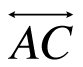and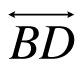.

3. Compare the slopes ofand. What do you notice?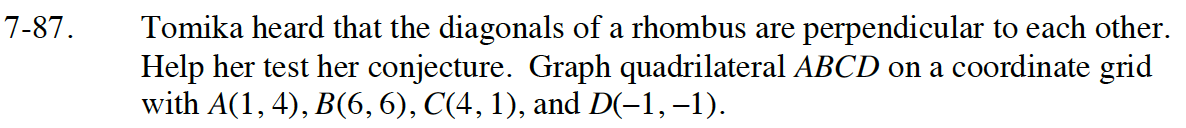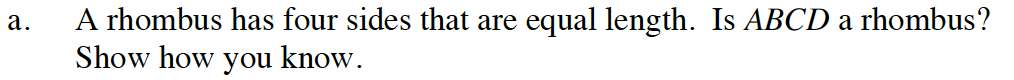Create slope triangles on each side. Visualize them using the eTool, then draw them on your paper.

Use the Pythagorean Theorem to calculate the length of each side. Are they the same? If so, the shape is a rhombus.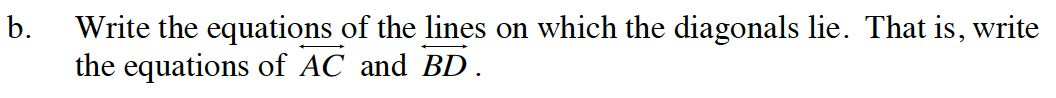Connect points A and C and points B and D. Use slope-intercept form (y = mx + b) to write the equations of the lines. Test your equations in the eTool to be sure they are correct.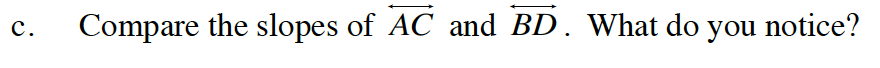What is true about the slopes of parallel and perpendicular lines?

Use the eTool below for help with parts (a-c).
Click the link at right for the full version of the eTool: Int1 7-87 HW eTool